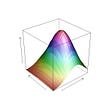The two most popular types of trading strategies are Momentum and Mean Reversion. You can read more about the Momentum Trading strategy in my post here. A Mean Reversion Trading Strategy involves betting that prices will revert back towards the mean or average, whereas, the Momentum Trading Strategy predicts prices will continue in the same direction.

A simplistic example of a mean reversion strategy is to buy a stock after it has had an unusually large fall in price. When a stock has seen a big drop, there’s usually a good chance that it will bounce back to a more normal level.

Pairs Trading is a trading strategy that matches a long position in one stock/asset with an offsetting position in another stock/asset that is statistically related. For example, consider 2 stocks in our portfolio: A and B, that are economically linked. Assuming, Company A sells mattresses and Company B sells pillows. Since, mattresses and pillows are combined and sold together, we can see how an increase in the sale of mattresses and pillows will affect both companies. Most of the time, we’ll see that the movement of the stocks A and B would be analogus. But incase we notice a pattern, where the stock movement starts to diverge, we will leverage our assumption about their economic ties and mean-reversion. We will assume that this divergence is temporary and in the long run, the two stock values will revert back to their original movement.

The Pairs Trading involves taking a long position(buy) the asset that is priced low. At the same time, the strategy involves taking a short position (sell) on the asset that is priced high. In the future, when the stocks revert back to their original movement, we can close our positions. Closing a position implies that we sell the asset that was previously bought and we buy the asset that was initially shorted.

There are benefits of pairs trading rather than trading a single stock. Pairs Trading reduces our exposure to the overall market. With Pairs Trading, we reduce some of the impact of large market movements. So even if both the stocks in your portfolio (Stock A, Stock B) are following a downward movement, we are only concerned with the relative difference between the pair of stocks. To describe this difference between the pair of stocks, we will understand the spread and the hedge ratio.

A hedge is an investment that is made with the intention of reducing the risk of adverse price movements in an asset. Normally, a hedge consists of taking an offsetting position in a related security.

A hedge ratio is the comparative value of an open position’s hedge to the aggregate size of the position itself. It is expressed as a decimal or fraction and is used to quantify the amount of risk exposure one has assumed through remaining active in an investment or trade.

The formula for the hedge ratio is:

`Hedge Ratio = Value of the Hedge / Total Position Value`

To explain this, consider we have a long position in Stock A and short position in Stock B. The positons are in a certain propotion so that overall positon remains constant throughout the time. In other words, the movements in our long and short position cancel each other out. The propotion that helps us create this neutral position is called hedge ratio. Hedge ratio can be calculated as 2 ways:

• Price Ratio: In this method, we divide the price of Stock B (B) by price of Stock A (A).
• Regression: The second way is to run a linear regression, where Stock A is the independent variable and Stock B is the depended variable. The coefficient of this regression is our hedge ratio.

Once we have the hedge ratio, we can calculate the Spread. Spread is the price of Stock B minus the product of hedge ratio and price of Stock A. This difference between the estimation and actual value is called the Spread.

# Correlation and Cointegration

## Correlation

Correlation is quantified by the correlation coefficient ρ, which ranges from -1 to +1. The correlation coefficient indicates the degree of correlation between the two variables. The value of +1 means there exists a perfect positive correlation between the two variables, -1 means there is a perfect negative correlation and 0 means there is no correlation.

A perfect positive correlation is when one variable moves in either up or down direction, the other variable also moves in the same direction with the same magnitude while a perfect negative correlation is when one variable moves in the upward direction, the other variable moves in the downward (i.e. opposite) direction with the same magnitude.

The correlation coefficient for the two variables is given by

`Correlation(X,Y) = ρ = COV(X,Y) / SD(X).SD(Y)`

where, cov (X, Y) is the covariance between X & Y while SD (X) and SD(Y) denotes the standard deviation of the respective variables.

If the correlation is high, say 0.8, traders may choose that pair for pairs trading. This high number represents a strong relationship between the two stocks. So if A goes up, the chances of B going up are also quite high. Based on this assumption a market neutral strategy is played where A is bought and B is sold; bought and sold decisions are made based on their individual patterns.

## Cointegration

The most common test for Pairs Trading is the Cointegration Test. Cointegration is a statistical property of two or more time-series variables which indicates if a linear combination of the variables is stationary. A series is said to be stationary when its mean, variance and co-variance are stable over time. Consider the scenario below, if Stock X and Stock Y have an economic link, then a linear combination of both (subtracting one from the other) may result in a time series that is stationary.

Here, a time series represented by I(1) implies that that particular time series if integrated of order 1, i.e. if we take a time difference of this time series, we will get a stationary series. When a series is stationary, it is also integrated of order 0, I(0).

Now, Spread = log(a) — nlog(b), where ‘a’ and ‘b’ are prices of stocks A and B respectively. For each stock of A bought, you have sold n stocks of B. If A and B are cointegrated then it implies that this equation above is stationary. A stationary process has very valuable features which are required to model Pairs Trading strategies. For instance, in this case, if the equation above is stationary, that suggests that the mean and variance of this equation remains constant over time. So if we start with ’n’, which is called the hedge ratio, so that spread = 0, the property of stationary implies that the expected value of spread will remain as 0. Any deviation from this expected value is a case for statistical abnormality, hence a case for pairs trading!

## Relation between Correlation and Cointegration

A strong correlation means that when Stock A moves up, Stock B moves up at the same time and vice versa. However, if the magnitude of movement of one stock is greater than the other, the two stocks can drift apart even though they are correlated.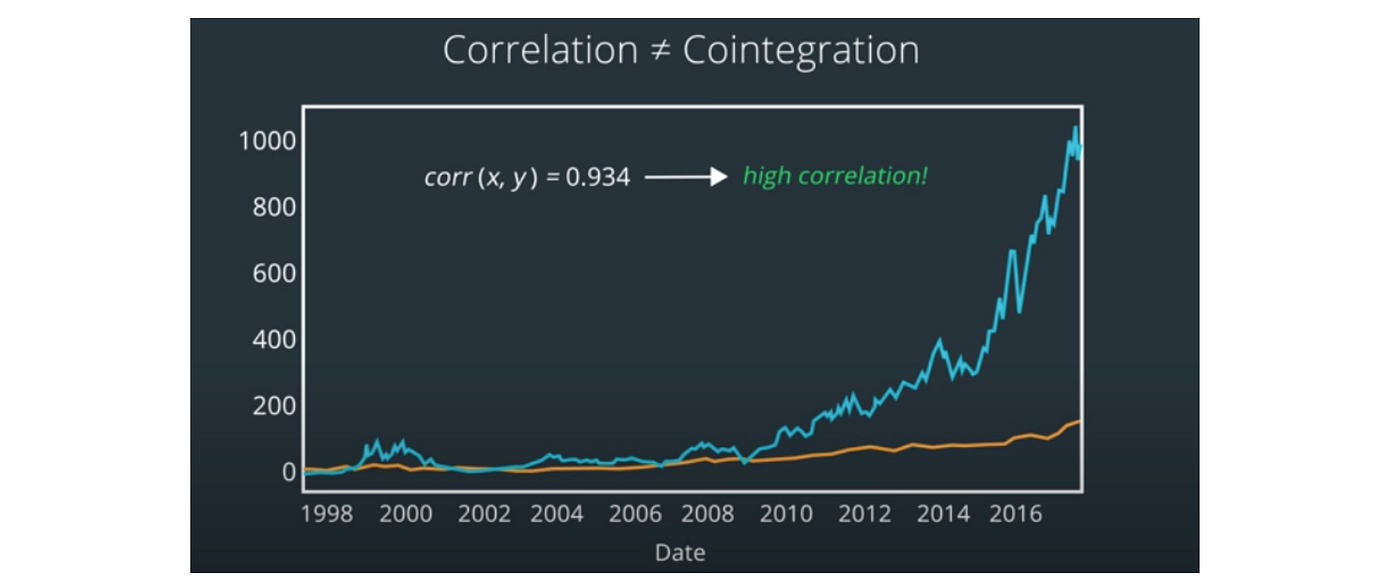Two correlated stocks may not be cointegrated. (Source: AI for Trading nano degree course on Udacity)

Cointegration means that over a range of days, the relative increase in Stock A is matched by the relative increase in Stock B. For Pairs Trading, we want to find stocks that are cointegrated. The method for checking if two series are cointegrated is called the Engle-Granger Test.

## Engle-Granger Test

The method to test if two series are co-integrated is called Engle-Granger Test.:

1. Get the hedge ratio from linear regression
2. Calculate spread and check if spread is stationary. To check if a spread is stationary, we use the Augmented Dickey-Fuller test. This test outputs a p-value. If this p-value is small (≤0.05), then we can assume that the spread is stationary, and hence the two are cointegrated.

# Jupyter Notebook

The Python code for checking if a pair of stocks are cointegrated or not can be found here:

--

--

--

## More from Purva Singh

Hi! I am a tech enthusiast currently working on leveraging language technologies to solve financial use-cases! View my work here: https://purvasingh96.github.io

Love podcasts or audiobooks? Learn on the go with our new app.

## Post-Negative Oil: The 1-Year Curvature as a measure of Forward Curve Evolution## K-Means Clustering simplified## Forecasting new COVID19 cases in Portugal using Gaussian Processes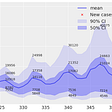## A friend of mine I went to high school with came round to visit me over the long weekend.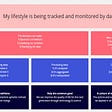## Sales Analysis## Fast Data Quality Framework on Great Expectations## Agolo Knowledge Graph API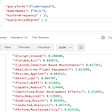## Purva Singh

Hi! I am a tech enthusiast currently working on leveraging language technologies to solve financial use-cases! View my work here: https://purvasingh96.github.io

## Value Analysis and Trading: Algorithmic Thought## Analyze Notional TVL market Correlation## Will Low-Code Replace Python for AI?## Multivariate Guassian Distribution